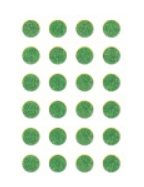Divide. Use objects, multiply, or subtract to solve.

Question 1.
8 ÷ 2 = ___
8 ÷ 2 = 4

Explanation:
I subtracted 2 from 8 until i reach 0
I subtracted 2 from 8 four times
So, 8 ÷ 2 = 4.

Question 2.
4 ÷ 4 = ___
4 ÷ 4 = 1

Explanation:
Any number divided by itself is 1
Four divided by four is 1
So, 4 ÷ 4 = 1.

Question 3.
4 ÷ 2 = ___
4 ÷ 2 = 2

Explanation:
I subtracted 2 from 4 until i reach 0
I subtracted 2 from 4 two times
So, 4 ÷ 2 = 2.

Question 4.
2 ÷ 2 = ___
2 ÷ 2 = 1

Explanation:
Any number divided by itself is 1
Two divided by two is 1
So, 2 ÷ 2 = 1.

Question 5.
16 ÷ 4 = ___
16 ÷ 4 = 4

Explanation:
I subtracted 4 from 16 until i reach 0
I subtracted 4 from 16 four times
So, 16 ÷ 4 = 4.

Question 6.
32 ÷ 4 = ___
32 ÷ 4 = 8

Explanation:
I subtracted 4 from 32 until i reach 0
I subtracted 4 from 32 eight times
So, 32 ÷ 4 = 8.

Question 7.
18 ÷ 2 = ___
18 ÷ 2 = 9

Explanation:
I subtracted 2 from 18 until i reach 0
I subtracted 2 from 18 nine times
So, 18 ÷ 2 = 9.

Question 8.
36 ÷ 4 = __
36 ÷ 4 = 9

Explanation:
I subtracted 4 from 36 until i reach 0
I subtracted 4 from 36 nine times
So, 36 ÷ 4 = 9.

Question 9.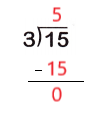Explanation:
Fifteen divided by three equals five
So, 15 ÷ 3 = 5.

Question 10.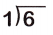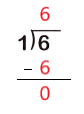Explanation:
Any number divided by 1 is the number itself
So, 6 ÷ 1 = 6.

Question 11.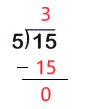Explanation:
Fifteen divided by five equals three
So, 15 ÷ 5 = 3.

Question 12.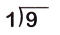Explanation:
Any number divided by 1 is the number itself
So, 9 ÷ 1 = 9.

Question 13.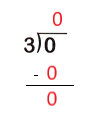Explanation:
Zero divided by any number is zero
So, 0 ÷ 3 = 0.

Question 14.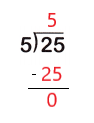Explanation:
Twenty five divided by five equals five
So, 25 ÷ 5 = 5.

Question 15.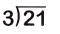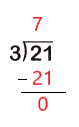Explanation:
Twenty one divided by three is seven
So, 21 ÷ 3 = 7.

Question 16.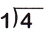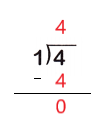Explanation:
Any number divided by 1 is the number itself
So, 4 ÷ 1 = 4.

Question 17.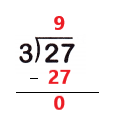Explanation:
Twenty seven divided by three is nine
So, 27 ÷ 3 = 9.

Question 18.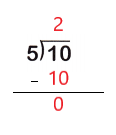Explanation:
Ten divided by five is two
So, 10 ÷ 5 = 2.

Question 19.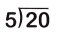Explanation:
Twenty divided by five is four
So, 20 ÷ 5 = 4.

Question 20.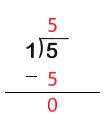Explanation:
Any number divided by 1 is the number itself
So, 5 ÷ 1 = 5.

Question 21.
Fill in the blanks. Use the 9 as the dividend and 3 as the divisor. Then solve.
______ ÷ ____ = ______
9 ÷ 3 = 3

Explanation:
I subtracted 3 from 9 until i reach 0
I subtracted 3 from 9 three times
So, 9 ÷ 3 = 3.

Divide to find the missing factor.

Question 22.
3 × ? = 21
______ ÷ ____ = ______
_____ × 7 = 21
21 ÷ 3 = 7
3 × 7 = 21

Explanation:
Division can help to multiply
3 x ? = 21
The division sentence with the given numbers
21 ÷ 3 = ?
21 ÷ 3 = 7
The quotient is the missing factor
So, 3 x 7 = 21.

Question 23.
? × 4 = 16
______ ÷ ____ = ______
_____ × 4 = 16
16 ÷ 4 = 4
4 × 4 = 16

Explanation:
Division can help to multiply
? x 4 = 16
The division sentence with the given numbers
16 ÷ 4 = ?
16 ÷ 4 = 4
The quotient is the missing factor
So, 4 x 4 = 16.

Multiply to find the quotient.

Question 24.
28 ÷ 4 = ?
_____ × ____ = _____
28 ÷ 4 = ____
4 × 7 = 28
28 ÷ 4 = 7

Explanation:
Multiplication can help to divide
28 ÷ 4 = ?
The multiplication equation with the given numbers
4 x ? = 28
4 x 7 = 28
So, 28 ÷ 4 = 7.

Question 25.
14 ÷ 2 = ___
_____ × ____ = _____
14 ÷ 2 = ____
2 × 7 = 14
14 ÷ 2 = 7

Explanation:
Multiplication can help to divide
14 ÷ 2 = ?
The multiplication equation with the given numbers
2 x ? = 14
2 x 7 = 14
So, 14 ÷ 2 = 7.

Question 26.
Read the division problem. Write the multiplication sentence you can use to solve it.
Mr. Newton is making 3 winter coats. Each coat must have an equal number of buttons. How many buttons should he sew on each coat if he has 12 buttons?
_________________
4 buttons

Explanation:
Mr. Newton is making 3 winter coats
Each coat must have an equal number of buttons
He has 12 buttons
Multiplication can help to divide
12 ÷ 3 = ?
The multiplication equation with the given numbers
3 x ? = 12
3 x 4 = 12
So, 12 ÷ 3 = 4
So, Mr. Newton should sew 4 buttons on each coat if he has 12 buttons.

Question 27.
Write the fact family that uses 4, 32, and 8.
_________ × ____ = _____
_________ × ____ = _____
_____ ÷ _____ = ____
_____ ÷ _____ = ____
8 x 4 = 32
4 x 8 = 32
32 ÷ 4 = 8
32 ÷ 8 = 4

Explanation:
Some multiplication and division sentences use the same numbers.
They make up a fact family
I wrote the fact family for the numbers 4, 32 and 8.

Question 28.
Faith picks 45 strawberries. She wants to give 5 strawberries each to 9 friends. Does she have enough strawberries?
Yes, Faith have enough strawberries

Explanation:
Faith picks 45 strawberries
She wants to give 5 strawberries each to 9 friends
Divide 45 by 5 to check whether Faith have enough strawberries to give 9 friends
45 ÷ 5 = ?
5 x ? = 45
5 x 9 = 45
So, Faith have enough strawberries to give 9 of his friends.

Question 29.
It takes 4 lemons to make 1 pitcher of lemonade. Ryan has 24 lemons. Ryan has 5 trays of ice. How many pitchers can Ryan make if he uses all the lemons? If you need to, draw a picture to help solve.